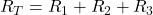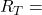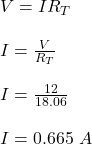## A 2.25 Ω resistor, a 5.90 Ω resistor, and a 9.91 Ω resistor are connected in series with a 12.0 V battery. What is the equivalent resistance

Question

A 2.25 Ω resistor, a 5.90 Ω resistor, and a 9.91 Ω resistor are connected in series with a 12.0 V battery. What is the equivalent resistance? Submit Answer Tries 0/20 What is the current in each resistor?

in progress 0
6 months 2021-08-17T07:29:53+00:00 1 Answers 13 views 0

(a) The equivalent resistance is 18.06 Ω

(b) The current in each resistor is 0.665 A

Explanation:

Given;

first resistor, R₁ = 2.25 Ω

second resistor, R₂ = 5.90 Ω

third resistor, R₃ = 9.91 Ω

Equivalent resistance;2.25 Ω +  5.90 Ω + 9.91 Ω18.06 Ω

The current in each resistor;

For series connection, current is the same in every resistor.

V = I(R₁ + R₂ + R₃)